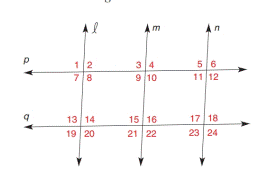Chapter 2.3, Problem 16E### Elementary Geometry for College St...

6th Edition
Daniel C. Alexander + 1 other
ISBN: 9781285195698

#### Solutions

Chapter
Section### Elementary Geometry for College St...

6th Edition
Daniel C. Alexander + 1 other
ISBN: 9781285195698
Textbook Problem
4 views

# In Exercise 7 to 16, name the lines (if any) that must be parallel under the given conditions.The bisectors of ∠ 9 and ∠ 21 are parallel.

To determine

To find:

The parallel lines.

Explanation

Given:

The given statement is,

The bisectors of 9 and 21 are parallel.

Approach:

(1) If two lines are cut by a transversal so that two corresponding angles are congruent, then these lines are parallel.

(2) If two parallel lines are cut by a transversal, then the pairs of corresponding angles on the same side of the transversal are supplementary.

Calculation:

The given statement is,

The bisectors of 9 and 21 are parallel.

Lines a and b are bisectors of 9 and 21 respectively.

Line a bisects 9 into w and x.

Line b bisects 21 into z and y.

Lines a and b are parallel and cut by transversal m

### Still sussing out bartleby?

Check out a sample textbook solution.

See a sample solution

#### The Solution to Your Study Problems

Bartleby provides explanations to thousands of textbook problems written by our experts, many with advanced degrees!

Get Started

#### Explain the difference between a matched-subjects design and a repeated-measures design.

Essentials of Statistics for The Behavioral Sciences (MindTap Course List)

#### Evaluate the integrals in Problems 1-32. 20.

Mathematical Applications for the Management, Life, and Social Sciences

#### In Exercises 1124, find the indicated limits, if they exist. 21. limxx2x21

Applied Calculus for the Managerial, Life, and Social Sciences: A Brief Approach

#### True or False: If f(x) 0 for all x in an interval I then f is increasing on I.

Study Guide for Stewart's Single Variable Calculus: Early Transcendentals, 8th

#### True or False: is a convergent series.

Study Guide for Stewart's Multivariable Calculus, 8th